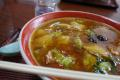# \$BM7:4\$R\$^\$o\$j?)F2\$N%T%j?I8^L\(B\$B\$d\$C\$Q\$j\$&\$^\$\$\$C\$F!#(B\$B@h=5Cf2Z\$=\$P(B\$B\$r(B \$B?)\$Y\$?\$H\$-\$K\$9\$4\$/\$\$\$\$=P\$7\$N9a\$j\$@\$C\$?\$N\$G4|BT\$7\$F%T%j?I!#(B \$B@lMQ\$K=P\$7\$r